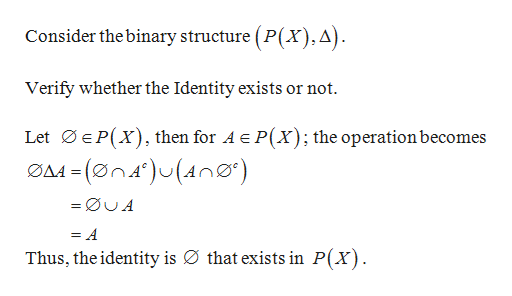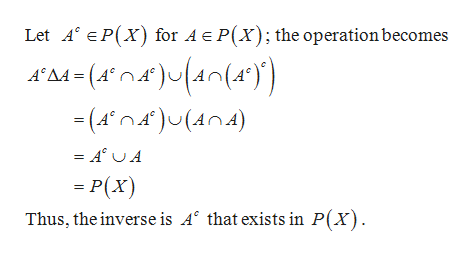Let X be a set and define the binary operation ∆ on the subsets of X by A∆B := (A∩Bc)∪(B∩Ac).Prove that the set P(X) of all subsets of X is a group under the operation ∆.

Question
1. Let X be a set and define the binary operation ∆ on the subsets of X by A∆B := (A∩Bc)∪(B∩Ac).

Prove that the set P(X) of all subsets of X is a group under the operation ∆.

Step 1

Let X be a set and define the binary operation ∆ on the subsets of X by

Step 2

Prove that the set P(X) of all subsets of X is a group under the operation ∆.

Proof:help_outlineImage TranscriptioncloseConsider the binary structure (P(x),A). Verify whether the Identity exists or not Let EP(X) then for A e P(X); the operationbecomes A -(øn4)(n) = =ØUA = A Thus, the identity is Ø that exists in P(X) fullscreen
Step 3

Now, verify whether the Inv...help_outlineImage TranscriptioncloseLet A EP(X) for AEP(X); the operation becomes A'A4 (A)4n(4°) (An)U(An4) P(x) Thus, the inverse is A that exists in P(x) fullscreen

Want to see the full answer?

See Solution

Want to see this answer and more?

Our solutions are written by experts, many with advanced degrees, and available 24/7

See Solution
Tagged in

Math